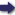(London :  Kegan Paul, Trench, Trübner & Co.,  1910.)

 Tools

## Search this bookPrev Page 25 Next``` CHAPTER LI. 25 divisor of 720, we get 2,160,000 solar days, 2,226,389 lunar days, 66,389 days of the adhimdsa months. If we, lastly, divide the universal solar, lunar, and civil days of a caturyuga, each kind separately, by the uni¬ versal adhimdsa months of a caturyuga, the quotient represents the numbers of days within which a whole adhimdsa month sums up, viz. in 976^-i-i-i-^ solar days, in ioo6-g%%%\ lunar days, and in 990-|-^f|- civil days. These are the elements of the computation of the adhimdsa, which we have worked out for the benefit of the following investigations. Kegarding the cause which necessitates the unardtra, Explanation lit. tloe days of the decrease, we have to consider the f ol- °(M(«4ra^."^ lowing. If we have one year or a certain number of years, and reckon for each of them twelve months, we get the corresponding number of solar months, and by multi¬ plying the latter by 30, the corresponding number of solar days. It is evident that the number of the lunar months or days of the same period is the same, plus an increase which forms one or several adhimdsa months. If we reduce this increase to adJiimdsa months due to the period of time in question, according to the relation between the universal solar months and the universal adhimdsa months, and add this to the months or days of the years in question, the sum represents the partial lunar days, i.e. those which correspond to the given number of years. This, however, is not what is wanted. What we want is the number of civil days of the given number of years which are less than the lunar days; for one civil day is greater than one lunar day. Therefore, in order to find that which is sought, we must subtract some¬ thing from the number of lunar days, and this element which must be subtracted is called Unardtra. The Unardtra of the partial lunar days stands in the same relation to the universal lunar days as the universal ```Prev Page 25 Next